# MAFS.8.EE.1.3Archived StandardExport Print
Use numbers expressed in the form of a single digit times an integer power of 10 to estimate very large or very small quantities, and to express how many times as much one is than the other. For example, estimate the population of the United States as 3 ×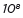and the population of the world as 7 ×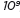, and determine that the world population is more than 20 times larger.
General Information
Subject Area: Mathematics
Domain-Subdomain: Expressions & Equations
Cluster: Level 1: Recall
Cluster: Work with radicals and integer exponents. (Major Cluster) -

Clusters should not be sorted from Major to Supporting and then taught in that order. To do so would strip the coherence of the mathematical ideas and miss the opportunity to enhance the major work of the grade with the supporting clusters.

Date of Last Rating: 02/14
Status: State Board Approved - Archived
Assessed: Yes
Test Item Specifications

• Assessment Limits :

N/A

• Calculator :

No

• Context :

Allowable

Sample Test Items (2)
• Test Item #: Sample Item 1
• Question:

The average mass of a giraffe is approximately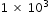kilograms. The average mass of a blue whale is approximately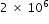.

About how many times more mass does a blue whale have than a giraffe?

• Difficulty: N/A
• Type: EE: Equation Editor

• Test Item #: Sample Item 2
• Question:

The average mass of an ant is approximately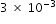grams. The average mass of a giraffe is approximately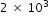kilograms.

About how many times more mass does a giraffe have than an ant?

• Difficulty: N/A
• Type: EE: Equation Editor

## Related Courses

This benchmark is part of these courses.
1205050: M/J Accelerated Mathematics Grade 7 (Specifically in versions: 2014 - 2015, 2015 - 2020, 2020 - 2022, 2022 and beyond (current))
1205070: M/J Grade 8 Pre-Algebra (Specifically in versions: 2014 - 2015, 2015 - 2022, 2022 and beyond (current))
1204000: M/J Foundational Skills in Mathematics 6-8 (Specifically in versions: 2014 - 2015, 2015 - 2022, 2022 and beyond (current))
7812030: Access M/J Grade 8 Pre-Algebra (Specifically in versions: 2014 - 2015, 2015 - 2018, 2018 - 2019, 2019 - 2022, 2022 and beyond (current))

## Related Access Points

Alternate version of this benchmark for students with significant cognitive disabilities.

## Related Resources

Vetted resources educators can use to teach the concepts and skills in this benchmark.

## Formative Assessments

Estimating Length Using Scientific Notation:

This lesson unit is intended to help you assess how well students are able to:

• Estimate lengths of everyday objects.
• Convert between decimal and scientific notation.
• Make comparisons of the size of numbers expressed in both decimal and scientific notation.

Type: Formative Assessment

Estimating Extreme Values:

Students are asked to estimate an extremely large and an extremely small number by writing it in the form a x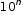.

Type: Formative Assessment

Order Matters:

Students are given pairs of numbers written in the form of an integer times a power of 10 and are asked to compare the numbers in each pair using the inequality symbols.

Type: Formative Assessment

How Many Times?:

Students are given pairs of numbers written in exponential form and are asked to compare them multiplicatively.

Type: Formative Assessment

Compare Numbers:

Students are given pairs of numbers written in scientific notation and are asked to compare them multiplicatively.

Type: Formative Assessment

## Lesson Plan

Estimating Length Using Scientific Notation:

This lesson unit is intended to help you assess how well students are able to estimate lengths of everyday objects, convert between decimal and scientific notation and make comparisons of the size of numbers expressed in both decimal and scientific notation.

Type: Lesson Plan

## Original Student Tutorial

Scientific Notation: Expressing Large Quantities:

Explore how to express large quantities using scientific notation in this interactive tutorial.

Type: Original Student Tutorial

Pennies to Heaven:

The goal of this task is to give students a context to investigate large numbers and measurements. Students need to fluently convert units with very large numbers in order to successfully complete this task. The total number of pennies minted either in a single year or for the last century is phenomenally large and difficult to grasp. One way to assess how large this number is would be to consider how far all of these pennies would reach if we were able to stack them one on top of another: this is another phenomenally large number but just how large may well come as a surprise.

Ant and Elephant:

In this problem students are comparing a very small quantity with a very large quantity using the metric system. The metric system is especially convenient when comparing measurements using scientific notations since different units within the system are related by powers of ten.

## Student Center Activity

Students can practice answering mathematics questions on a variety of topics. With an account, students can save their work and send it to their teacher when complete.

Type: Student Center Activity

## MFAS Formative Assessments

Compare Numbers:

Students are given pairs of numbers written in scientific notation and are asked to compare them multiplicatively.

Estimating Extreme Values:

Students are asked to estimate an extremely large and an extremely small number by writing it in the form a x.

How Many Times?:

Students are given pairs of numbers written in exponential form and are asked to compare them multiplicatively.

Order Matters:

Students are given pairs of numbers written in the form of an integer times a power of 10 and are asked to compare the numbers in each pair using the inequality symbols.

## Original Student Tutorials Mathematics - Grades 6-8

Scientific Notation: Expressing Large Quantities:

Explore how to express large quantities using scientific notation in this interactive tutorial.

## Student Resources

Vetted resources students can use to learn the concepts and skills in this benchmark.

## Original Student Tutorial

Scientific Notation: Expressing Large Quantities:

Explore how to express large quantities using scientific notation in this interactive tutorial.

Type: Original Student Tutorial

Pennies to Heaven:

The goal of this task is to give students a context to investigate large numbers and measurements. Students need to fluently convert units with very large numbers in order to successfully complete this task. The total number of pennies minted either in a single year or for the last century is phenomenally large and difficult to grasp. One way to assess how large this number is would be to consider how far all of these pennies would reach if we were able to stack them one on top of another: this is another phenomenally large number but just how large may well come as a surprise.

## Student Center Activity

Students can practice answering mathematics questions on a variety of topics. With an account, students can save their work and send it to their teacher when complete.

Type: Student Center Activity

## Parent Resources

Vetted resources caregivers can use to help students learn the concepts and skills in this benchmark.